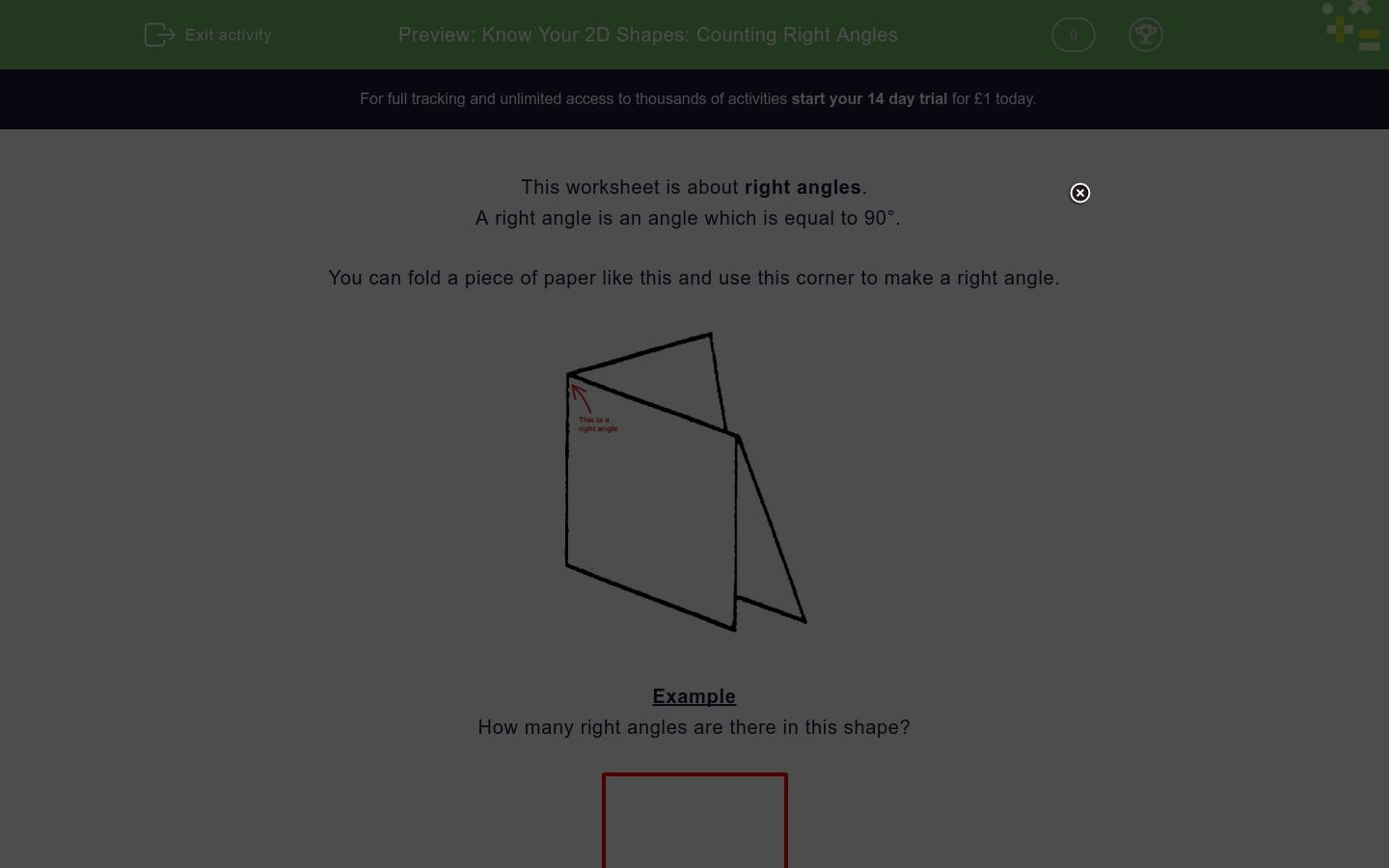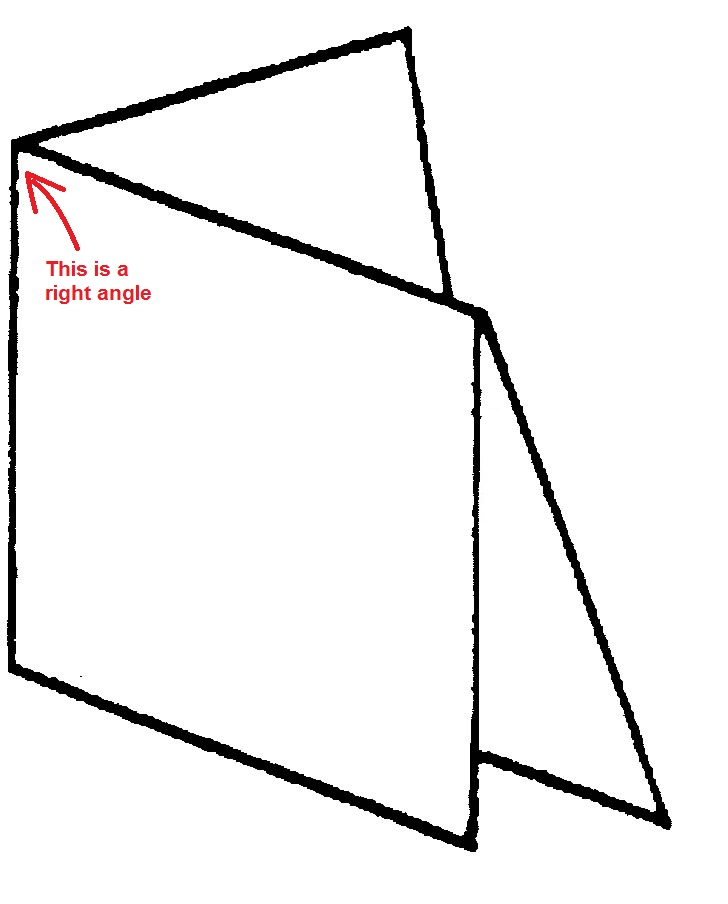# Know Your 2D Shapes: Counting Right Angles

In this worksheet,students count the number of right angles in the 2D shapes shown.Key stage:  KS 2

Curriculum topic:   Geometry: Properties of Shapes

Curriculum subtopic:   Identify Right Angles

Difficulty level:### QUESTION 1 of 10

This worksheet is about right angles.

A right angle is an angle which is equal to 90°.

You can fold a piece of paper like this and use this corner to make a right angle.Example

How many right angles are there in this shape?There are 4 right angles, shown in blue.

Want to understand this further and learn how this links to other topics in maths?
Why not watch this video?

How many right angles are there in this shape?5

0

6

How many right angles are there in this shape?0

2

3

How many right angles are there in this shape?1

2

3

How many right angles are there in this shape?4

2

3

How many right angles are there in this shape?4

2

3

How many right angles are there in this shape?4

2

3

How many right angles are there in this shape?5

2

0

How many right angles are there in this shape?5

2

0

How many right angles are there in this shape?3

2

5

How many right angles are there inside this shape?6

2

0

• Question 1

How many right angles are there in this shape?0
EDDIE SAYS
None of the angles are right angles.
• Question 2

How many right angles are there in this shape?0
EDDIE SAYS
None of the angles are right angles.
• Question 3

How many right angles are there in this shape?1
EDDIE SAYS• Question 4

How many right angles are there in this shape?4
EDDIE SAYS• Question 5

How many right angles are there in this shape?2
EDDIE SAYS• Question 6

How many right angles are there in this shape?4
EDDIE SAYS• Question 7

How many right angles are there in this shape?0
EDDIE SAYS
There are no right angles in this pentagon.
• Question 8

How many right angles are there in this shape?0
EDDIE SAYS
There are no right angles in this curved shape. It is called an oval or an ellipse.
• Question 9

How many right angles are there in this shape?3
EDDIE SAYS• Question 10

How many right angles are there inside this shape?2
EDDIE SAYS---- OR ----